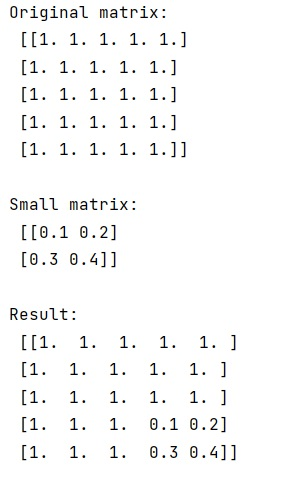# How to replace sub part of matrix by another small matrix in NumPy?

Learn, how can we replace sub part of matrix by another small matrix in NumPy?
Submitted by Pranit Sharma, on March 06, 2023

## NumPy - Replacing sub part of matrix by another small matrix

Suppose that we are given a numpy matrix and we need to replace a small part of this matrix with another small matrix.

Replacing sub part of matrix by another small matrix can be simply done using the indexing, we just need to select the piece of the matrix that we need to replace which must be of the same shape as the small matrix, and assign this piece of the matrix as the small matrix.

Let us understand with the help of an example,

## Python code to replace sub part of matrix by another small matrix in NumPy

```# Import numpy
import numpy as np

# Creating an array
arr = np.ones((5,5))

# Display Original matrix
print("Original matrix:\n",arr,"\n")

# Creating a small matrix
small = np.array([[ 0.1,  0.2],[ 0.3,  0.4]])

# Display small matrix
print("Small matrix:\n",small,"\n")

# replacing a part of big matrix with small matrix
arr[3:5, 3:5] = small

# Display result
print("Result:\n",arr)
```

Output:Languages: » C » C++ » C++ STL » Java » Data Structure » C#.Net » Android » Kotlin » SQL
Web Technologies: » PHP » Python » JavaScript » CSS » Ajax » Node.js » Web programming/HTML
Solved programs: » C » C++ » DS » Java » C#
Aptitude que. & ans.: » C » C++ » Java » DBMS
Interview que. & ans.: » C » Embedded C » Java » SEO » HR
CS Subjects: » CS Basics » O.S. » Networks » DBMS » Embedded Systems » Cloud Computing
» Machine learning » CS Organizations » Linux » DOS
More: » Articles » Puzzles » News/Updates